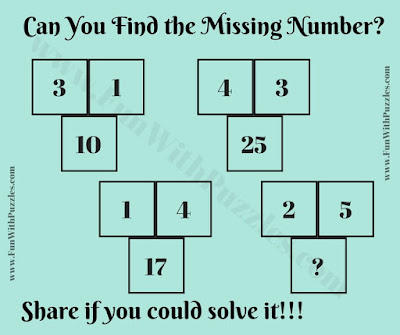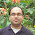Saturday, March 31, 2018

Here is test of your IQ. Lets see how much time you will take to solve this tricky IQ Question. In this tricky IQ question, there are some groups of three numbers which are related to each other. Can you find this relationship among these three numbers and then solve for the missing number?Can you find the missing Number?

Answer to this tricky IQ Question can be viewed by clicking on button. Please do give your best try before looking at the answer.

1.Shouldnt the answer be: 29
2 ×2 = 4 then
5x5 = 25 so therefore
The sum being 29

1.You are right. Answer should be 29. We have updated the correct answer now.
Thanks for finding this error.
Do let us know in case anywhere on this website you found similar error?

A comment doesn't cost a thing. Please drop a comment below to boost the author's morale.